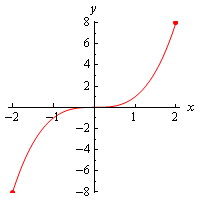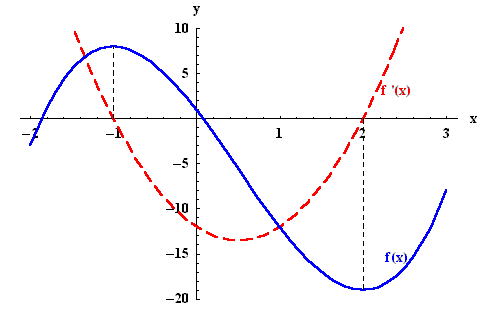×#### Thank you for registering.

One of our academic counsellors will contact you within 1 working day.

Click to Chat

1800-1023-196

+91-120-4616500

CART 0

• 0

MY CART (5)

Use Coupon: CART20 and get 20% off on all online Study Material

ITEM
DETAILS
MRP
DISCOUNT
FINAL PRICE
Total Price: Rs.

There are no items in this cart.
Continue Shopping```Applications of DerivativesAs we have discussed earlier that derivative is an important topic of calculus, similarly Applications of Derivatives also holds great importance as it fetches many direct questions in the IT JEE. With a bit of practice it is very easy to master this topic and there are some fixed patterns of questions asked from this topic. This section is the most scoring part of the JEE Mathematics syllabus.

This chapter covers various important topics like:

Motion in Straight Line

Geometrical Meaning of Derivative at Point

Monotonicity

Concept of Local Maxima and Local Minima

Global Maxima and Minima

Rolle Theorem and Lagrange Mean Value Theorem

Solved Examples of Applications of Derivatives

We just give here an outline of some of the topics as they will be discussed in detail in the coming sections:

Geometrical Interpretation of derivative

Let AB be the secant line passing through the points (x, f(x)) and (x +? x, f(x+?x)). If B→A i.e. ?x approaches zero, the secant approaches the tangent at (x, f(x)). Hence, when ?x approaches zero, the slope of the tangent is the limit of the slope of the secant.Hence, the derivative f'(x) can be interpreted as the slope of the tangent at point (x, y) on the graph of function y = f(x). From this interpretation, we get the following results:

(i) When a function is increasing on some interval, it is obvious that the slope of the tangent is positive at every point of that interval due to which its derivative is positive.

(ii) Similarly, in case a function is decreasing on some interval, the derivative is negative as the slope of the tangent is negative at every point of the interval.

Rolle's Theorem. Let f be a function which is differentiable on the closed interval [a, b]. If f(a) = f(b) then there exists a point c in (a, b) such that f '(c) = 0.

Mean Value Theorem. Let f be a function which is differentiable on the closed interval [a, b]. Then there exists a point c in (a, b) such that

f'(c) = {f(b)-f(a)}/ (b-a)

Maxima and Minima

There is a very slight difference between local maxima/minima and global maxima/minima. But it is very important to note that because this topic fetches various questions in the JEE.

Local Maximum: A function f is said to have a local maximum (also termed as relative maximum) at x=a if

f(x) ≤ f(c) , for every x in some open interval around x=c.

Local Minimum:  A function f is said to have a relative minimum or a local minimum around x=c if

f(x) ≥ f(c) , for every x in some open interval around x=a.

Global Maximum: A function f is said to have a global maximum (also termed as absolute maximum) at x=a if

f(x) ≤ f(c) , for every x in the domain under consideration

Global Minimum:  A function f is said to have a absolute minimum or a global minimum around x=c if

f(x) ≥ f(c) , for every x in the whole domain under consideration.

Illustration:

Find the absolute extrema and relative extrema for the following functions.

f(x) = x3 on [-2,2].

Solution:

We first draw the graph of the function so that the picture becomes clear.Substituting x=2 in the given function we get the function has absolute maximum of eight, while absolute minimum is -8 and occurs at x=-2. It is clear that there is no relative extrema.

Monotonicity

A function f defined on a subset of real numbers is said to be monotonic if it preserves the order. Mathematically, it is written as

For all x and y, such that x≤ y, if f(x) ≤ f(y), then the function f is said to be monotonically increasing, increasing or non-decreasing.

Similarly, for x≤y, if f(x) ≥ f(y), then the function is monotonically decreasing, decreasing or non-increasing i.e. it reverses the order.

You may refer the Past Papers to get an idea about the types of questions asked.

Illustration:

Consider the function f(x) = 2x3-3x2-12x+1.

Since f(x) is a polynomial function so it is continuous and differentiable everywhere. Hence, finding the derivative we get

f(x)= 6x2-6x-12 =6(x-2)(x+1).
So we haveHence, f(x) is increasing on the intervals (- ∞, -1] and [2,∞ ) and decreasing on the interval [-1,2].This video will provide you further clarification

Illustration 1: The function y = 2x2 – log |x| is monotonically increasing for values of x ≠ 0 satisfying the inequalities ……. and monotonically decreasing for values of x satisfying…..

Solution: The given function is y = 2x2 – log |x|.

x ∈ (-1/2, 0) ∪ (1/2, ∞), x ∈ (-∞ , -1/2) ∪ (0, ½)

Here, y = 2x2 – log x         , x >0

2x2 – log (-x)   , x < 0

So this implies

dy/dx = 4x – 1/x, x >0

= 4x – 1/x, x < 0

Hence, dy/dx = 4x2 – 1/x, x ∈ R – {0}

= (2x -1) (2x +1)/x

Therefore, it is increasing when x ∈(-1/2, 0) ∪ (1/2, ∞)

And decreasing when x ∈ (-∞, -1/2) ∪ (0, ½).

Illustration 2: The maximum value of

(cos α1). (cos α2)…. (cos αn), under the restrictions 0 ≤ α1, α2,… , αn ≤ π/2

(cot α1). (cot α2)…. (cot αn) = 1 is

1. 1/2n/2                                                            2. 1/2n

3. 1/2n                                                            4. 1

Solution: Given cot α1. cot α2….....cot αn = 1

Then, cos α1/sin α1 . cos α2/sin α2 . cos α3/sin α3 ….. cos αn/sin αn = 1.

Hence, cos α1. cos α2…. cos αn = k …….. (1)

and sin α1. sin α2……. sin αn = k …….. (2)

by multiplying equations (1) and (2) we get,

(cos α1. cos α2…… cos αn) x (sin α1. sin α2……. sin αn) = k2

Then k2 = 1/ 2.2.2…….. n times . (2 sin α1. cos α1) (2 sin α2. cos α2) (2 sin α3. cos α3)…………  (2 sin αn. cos αn)

Hence, k2 = 1/2n (sin 2α1).(sin 2α2)…….. (sin 2αn)

≤ 1/2n sin 2αi ≤ 1 for all 1≤ i ≤ n

Hence, k ≤ 1/2n/2. Hence the correct option is (1).

Illustration 3: If f(x) = xa log x and f(0) = 0, then the value of a for which the Rolle’s theorem can be applied in [0,1] is

1. -2                                                        2. -1

3. 0                                                         4. ½

Solution: In order to satisfy the Rolle’s Theorem, the function needs to be continuous in [0, 1].

So, lim x→0+ f(x) = f(0)

lim x→0+ log x/ x-a = 0.

lim x→0+ (1/x)/-ax-a-1 = 0.

lim x→0+ xa/ a = 0.

This clearly implies that a > 0.

Now, ∀ a > 0, f(x) is differential in (0, 1) and f (1) = 0 = f (0).

To read more, Buy study materials of Applications of Derivatives comprising study notes, revision notes, video lectures, previous year solved questions etc. Also browse for more study materials on Mathematics here.
```### Course Features

• 731 Video Lectures
• Revision Notes
• Previous Year Papers
• Mind Map
• Study Planner
• NCERT Solutions
• Discussion Forum
• Test paper with Video Solution